### IS Curve Equation Application Two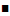Suppose that initially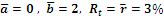. Then there is a demand shock to consumption whereand the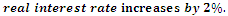Then short-run output will rise by 1%     See page 246-247 (of Jones Macro Econ)Starting a bit into the IS curve calculation, we know that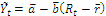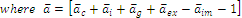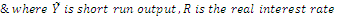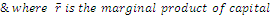Plugging in our given numbers (and I suppose assuming that consumption increasing by 5% is to mean that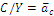increases by 5%)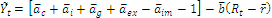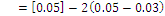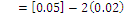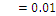The short-run economy will rise by 1%.
Comments